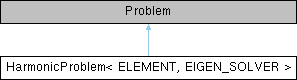HarmonicProblem< ELEMENT, EIGEN_SOLVER > Class Template Reference

1D Harmonic problem in unit interval. More...

Inheritance diagram for HarmonicProblem< ELEMENT, EIGEN_SOLVER >:## Public Member Functions

HarmonicProblem (const unsigned &n_element)
Constructor: Pass number of elements and pointer to source function. More...

~HarmonicProblem ()
Destructor (empty) More...

void solve (const unsigned &label)
Solve the problem. More...

void doc_solution (const unsigned &label)
Doc the solution, pass the number of the case considered, so that output files can be distinguished. More...

## Detailed Description

template<class ELEMENT, class EIGEN_SOLVER>
class HarmonicProblem< ELEMENT, EIGEN_SOLVER >

1D Harmonic problem in unit interval.

Definition at line 268 of file harmonic.cc.

## ◆ HarmonicProblem()

template<class ELEMENT , class EIGEN_SOLVER >
 HarmonicProblem< ELEMENT, EIGEN_SOLVER >::HarmonicProblem ( const unsigned & n_element )

Constructor: Pass number of elements and pointer to source function.

Constructor for 1D Harmonic problem in unit interval. Discretise the 1D domain with n_element elements of type ELEMENT. Specify function pointer to source function.

Definition at line 295 of file harmonic.cc.

## ◆ ~HarmonicProblem()

template<class ELEMENT , class EIGEN_SOLVER >
 HarmonicProblem< ELEMENT, EIGEN_SOLVER >::~HarmonicProblem ( )
inline

Destructor (empty)

Definition at line 276 of file harmonic.cc.

## ◆ doc_solution()

template<class ELEMENT , class EIGEN_SOLVER >
 void HarmonicProblem< ELEMENT, EIGEN_SOLVER >::doc_solution ( const unsigned & label )

Doc the solution, pass the number of the case considered, so that output files can be distinguished.

Doc the solution in tecplot format. Label files with label.

Definition at line 334 of file harmonic.cc.

## ◆ solve()

template<class ELEMENT , class EIGEN_SOLVER >
 void HarmonicProblem< ELEMENT, EIGEN_SOLVER >::solve ( const unsigned & label )

Solve the problem.

Solve the eigenproblem.

Definition at line 356 of file harmonic.cc.

Referenced by main().

The documentation for this class was generated from the following file: